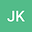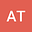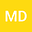•••Infiltration processes in fractured-porous media remain a crucial, yet not very well understood component of recharge and vulnerability assessment. Under partially-saturated conditions flows in fractures, percolating fracture networks and fault zones contribute to the fastest spectrum of infiltration velocities via preferential pathways. Specifically, the partitioning dynamics at fracture intersections determine the magnitude of flow fragmentation into vertical and horizontal components and hence the bulk flow velocity and dispersion of fracture networks. In this work we derive an analytical solution for the partitioning processes based on smoothed particle hydrodynamics simulations and laboratory studies. The developed transfer function allows to efficiently simulate flow through arbitrary long wide aperture fracture networks with simple cubic structure via linear response theory and convolution of a given input signal. We derive a non-dimensional bulk flow velocity ($\widetilde{v}$) and dispersion coefficient ($\widetilde{D}$) to characterize the system in terms of dimensionless horizontal and vertical time scales $\tau_m$ and $\tau_0$. The dispersion coefficient is shown to strongly depend on the horizontal time scale and converges towards a constant value of $0.08$ within reasonable ranges for the fluid and geometrical parameters, while the non-dimensional velocity exhibits a characteristic $\widetilde{v} \sim \tau_m^{-1/2}$ scaling. Given that hydraulic information is often only available at limited places within (fractured-porous) aquifer system, such as boreholes or springs, our study intends to provide a rudimentary analytical concept to potentially reconstruct internal fracture network geometries from external boundary information, e.g., the dispersive properties of discharge (groundwater level fluctuations).